#### 5.2.3Simulation Results

The first goal of this study was to understand the reasons for the stress discrepancy between the TSV and the full-plate samples. Therefore, the following configurations were considered for simulation: TSV without scallops, TSV with scallops, and TSV with weak adhesion. The first case was performed to control the hypothesis, whereas the other two cases were used for an evaluation of each particular feature on stress. The geometry of the scallops has been identified as the main cause for stress reduction inside the tungsten layer, as shown in Fig. 5.7. Although the weak adhesion has introduced some relaxation (17%), it superimposes to a stress state already reduced by the geometry. Moreover, the in-plane stress on tungsten is not equal in every direction in the presence of scallops. Krauss et al. were unaware of this fact during their measurements and assumed an in-plane equibiaxial condition for the stress . Nevertheless, this possibility is discussed in their work. Consequently, the reported stress by Krauss et al. does not fully characterize the state in the tungsten layer, but rather the average normal stress in the vertical direction, according to their described experimental setup.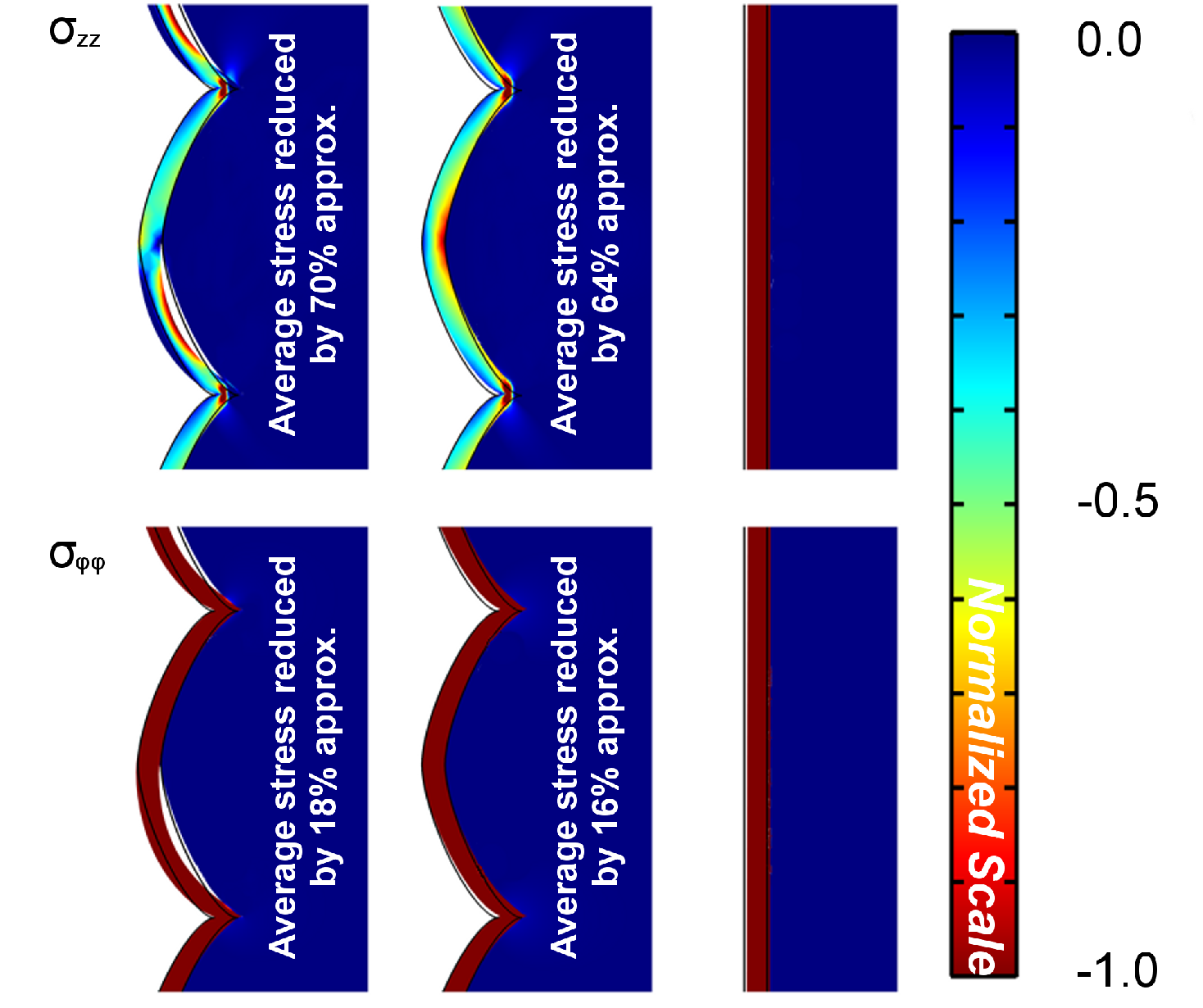Figure 5.7.: Stress distribution along the TSV in the vertical (top) and tangential direction (bottom) considering vias with weak adhesion (left), no weak adhession (middle) and without scallops (right). The stress is strongly reduced in the vertical direction, mainly due to the scalloped geometry. The weak adhesion induces a small reduction, but it is not the main mechanism. Along the tangential direction, the scalloped geometry is not so evident, hence the stress reduction is very small.

The double stack structure was simulated under the same conditions as the single stack, except that the weak adhesion case was not considered, as shown in Fig. 5.8. Since the equibiaxial stress assumption is not valid in the presence of scallops, the simulated and experimental stress results have not been compared. Instead, the strain was compared, which is a direct measure and free of any assumptions.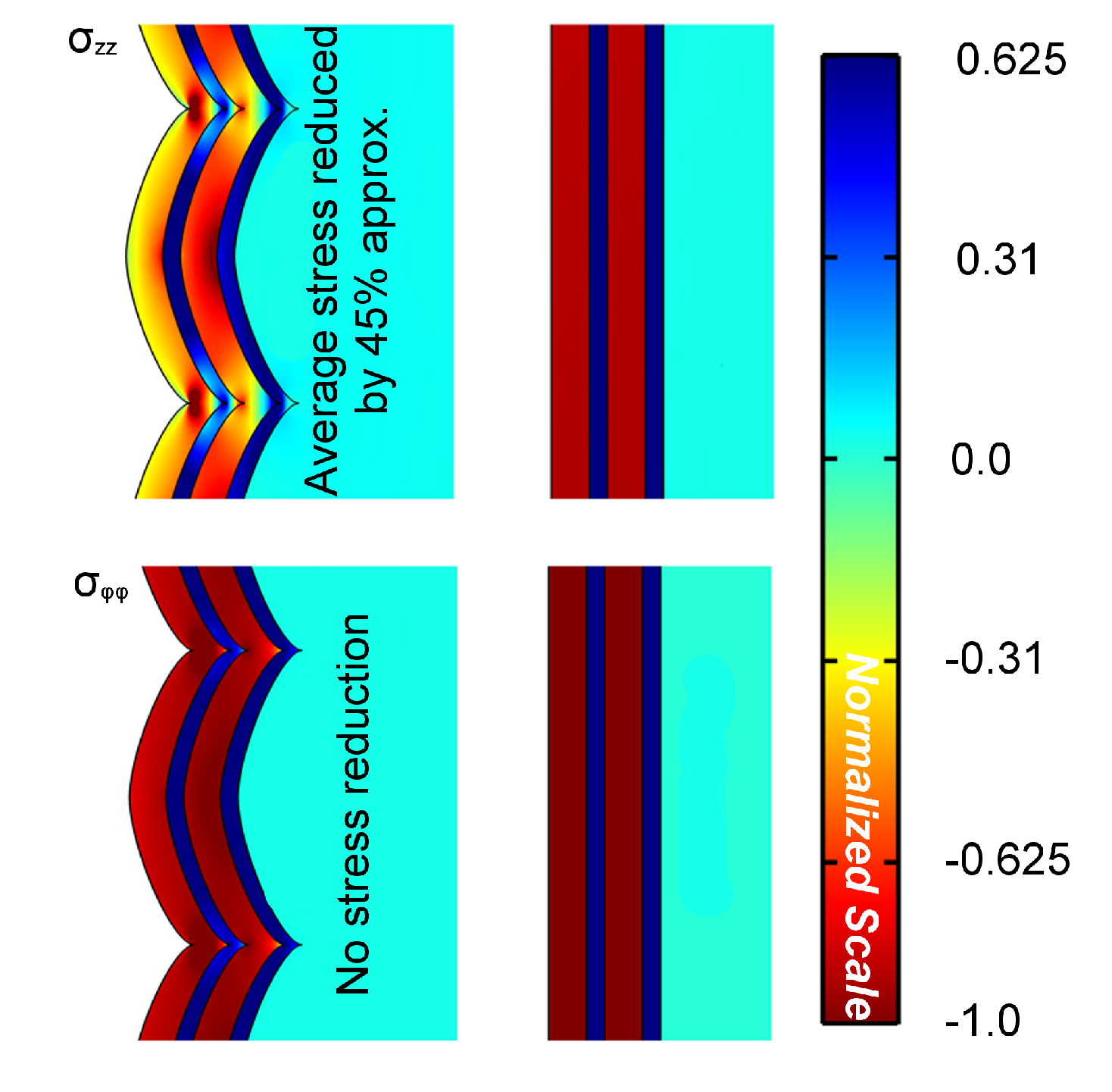Figure 5.8.: The same behavior for the stress is found in double stack structures. However, the reduction due to the scalloped sidewall is slightly smaller when compared to single stack TSVs.

The average simulated strain of 0.00169 in the vertical direction was in reasonable agreement with the measured strain of 0.00111  for the tungsten layer. The intrinsic stress on the Ti/TiN layers was ignored due to the lack of experimental data. However, simulations considering a compressive stress in the Ti/TiN layers resulted in an average strain of 0.00127 in the tungsten layer. Hence, the absence of the intrinsic stress on Ti/TiN can justify the difference between simulated and observed values.

The rigidity characteristic of the scallops in the vertical direction modifies the stress distribution on TSV films. The tensile stress induces an inward movement in the region of valleys between scallops, shown in Fig. 5.9. This leads to a relief of the stress along the via, since the material finds a favorable point to stretch. Consequently, the average normal stress in the vertical direction in tungsten is reduced, causing the difference between TSV and full-plate samples. However, in the tangential direction there is almost no stress reduction. Along this axis the geometry is not modified by the scallops. Therefore, the initial load faces a similar scenario in the presence or absence of scallops.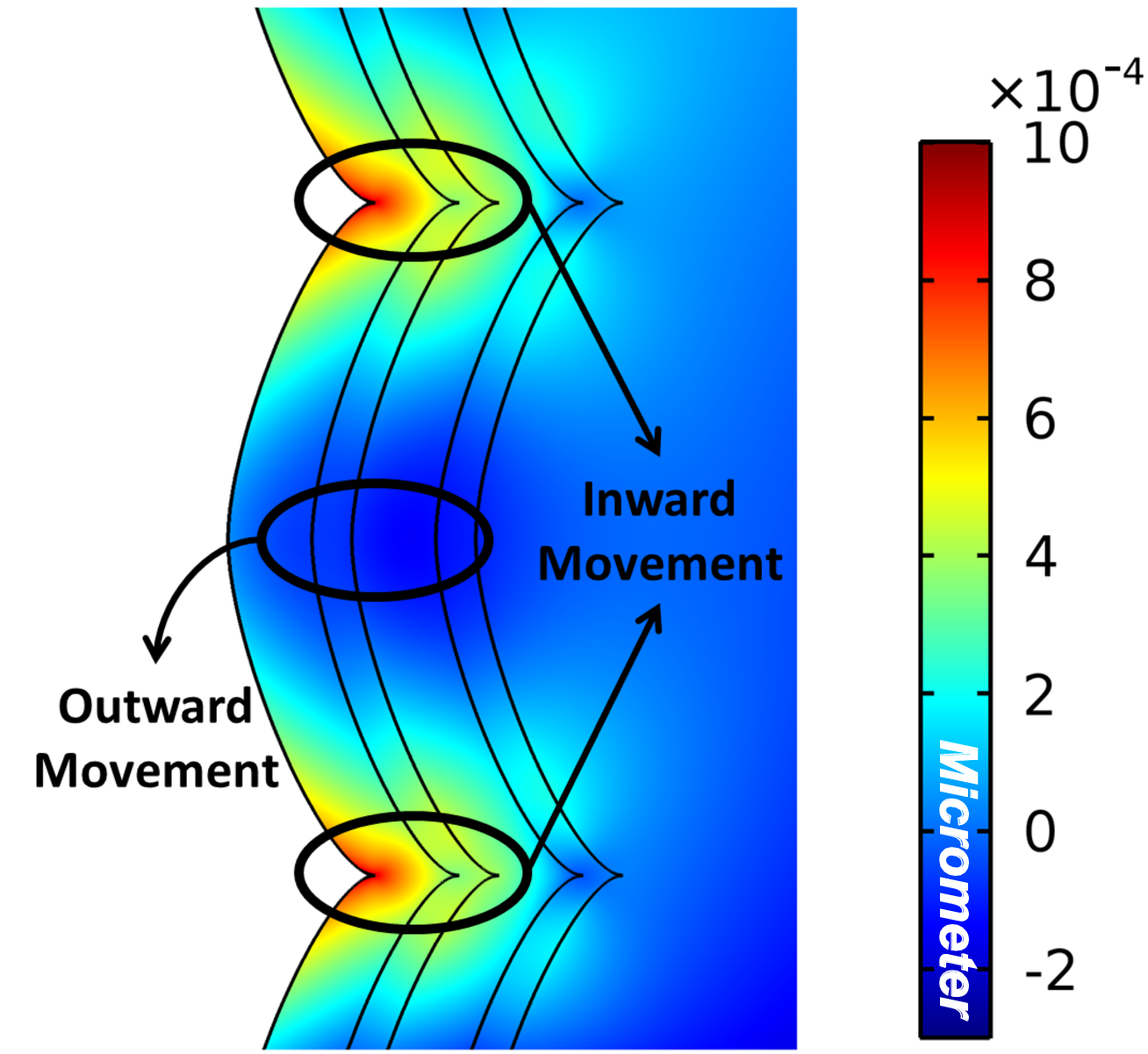Figure 5.9.: Displacement in the radial direction. The scallop creates points which ease the movement of the metal, relaxing the overall stress in the metal layer.

Additional insight concerning reliability is gathered from an analysis of the von Mises stress. The mean values for structures with and without scallops are very similar and no significant difference was detected. Hence, the full-plate sample measurements could be used safely to determine the average von Mises stress. However, the mean value of the von Mises stress did not suffice when the scallops were present due to the modified stress distribution. Fig. 5.10 outlines the accumulation stress points. The von Mises stress can reach peaks of stress up to 3 times higher than those experienced on full-plate samples. However, such high values could be the result of the sharp transition between the Bézier curves used to represent the scallops (singularity points). Even if the sharp transitions, observed with Bézier curves, were removed, there is still a pinched transition region between scallops. There, points of pinch-off are a region of potential failure, as increased stress could lead to a fracture in the metal.

Regarding scallop dimensions, the stress magnitude behavior depends on the scallops height and width, as shown in Fig. 5.11. As the height increases, the stress increases very rapidly until a peak is reached, then it drops slowly toward a saturation value. For small heights most of the scallop still retains its flat geometry, impeding material expansion and leading to an increased stress. This scenario persists until the scallop reaches a height which favors the curvy geometry and material expansion (flat characteristic is no longer dominant), resulting in stress relaxation. The peak stress in Fig. 5.11 is defined by this change in the scallop geometry. As the scallops’ widths are increased, the peaks broaden with a linear dependence, both in height and width directions. This peak movement is a consequence of the fact that wide scallops need higher heights to lose their flat behavior.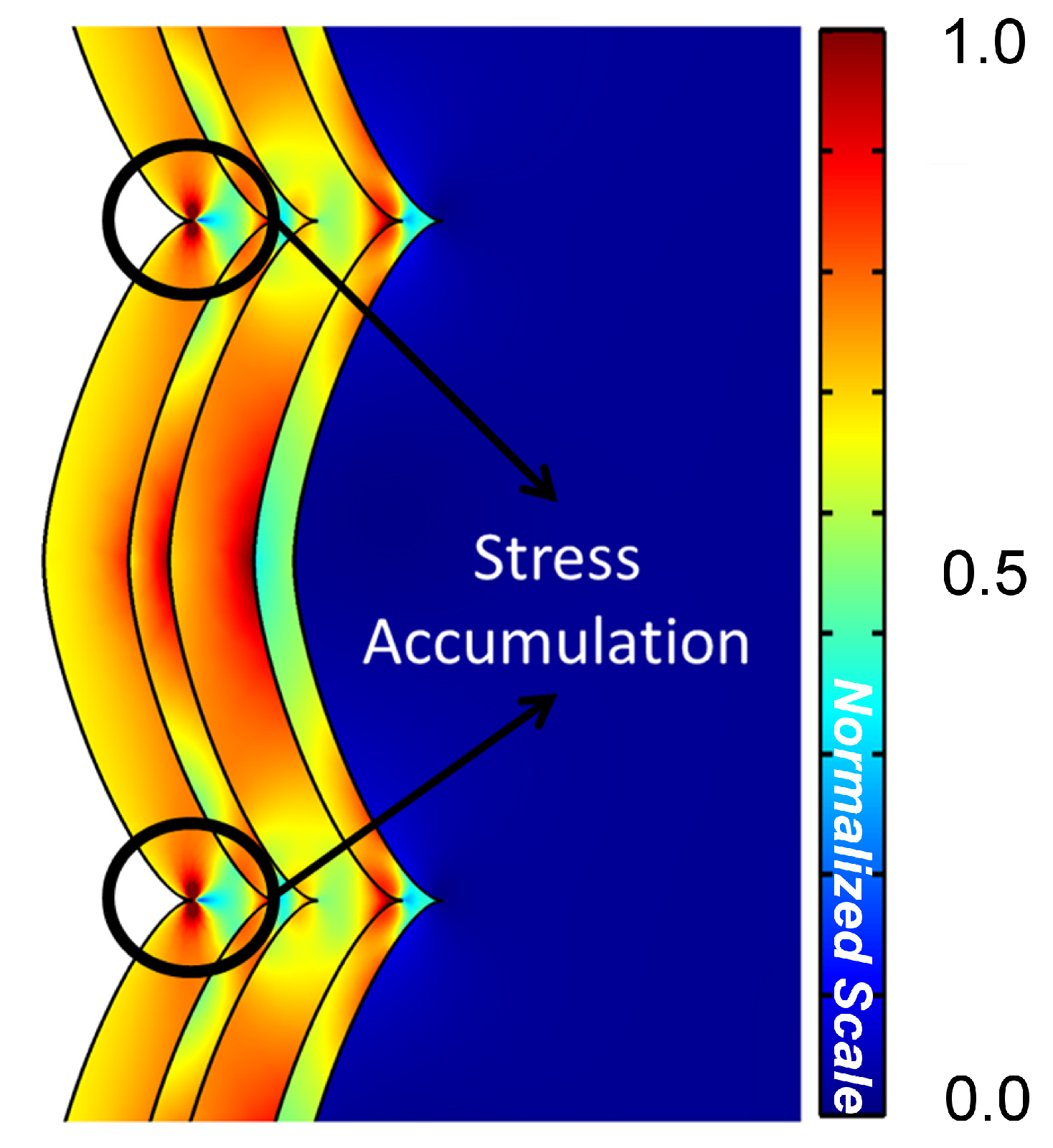Figure 5.10.: Points of high stress in the scallops. Although there is an overall reduction of the stress in the metal layers, the scallops create points of stress accumulation, which can easily surpass the average layer stress.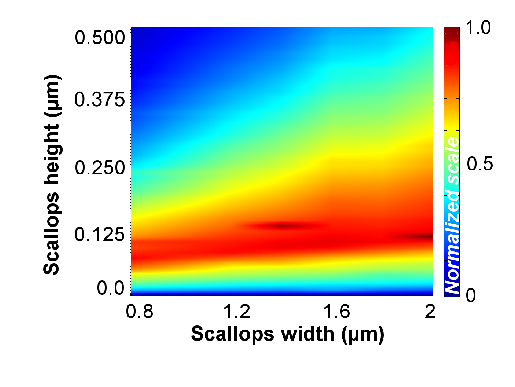Figure 5.11.: Maximum von Mises stress variation dependence on the height and width of the TSV sidewall scallops.